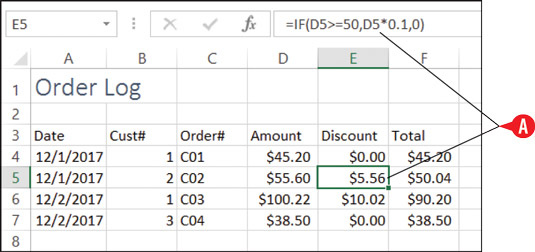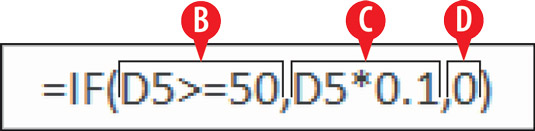##### Office 2016 at Work For DummiesThe IF function determines whether or not a condition is true, and then perform different actions in Excel 2016 based on that answer. IF is only one of many logical functions that Excel provides; see the list on the Logical button on the Formulas tab for others. For example:

• Suppose a customer gets a 10 percent discount if he spends more than \$50. You could use the IF function to determine whether his order amount qualifies.The amount of discount is determined using an IF function.

An IF function typically contains three arguments: condition, value_if_true, and value_if_false. Like all arguments, they are separated by commas.

• The condition in this example is D5>=50. In other words, is the value in D5 greater than or equal to 50?

• The value_if_true in this example is calculated by multiplying D5 by 0.1 (in other words, calculating 10 percent of it).

• The value_if_false in this example is zero (0).IF function with three arguments." width="535"/>
An IF function with three arguments.

The condition argument is the only required one. If you omit the value_if_true argument, the function returns 1 if the condition is true and 0 if the condition is false. If you omit the value_if_false argument, a value of 0 is assumed for it. Therefore in the above example, technically you wouldn’t have to include the value_if_false argument, since zero was the desired result anyway.

If you want to combine a SUM operation with an IF condition, you can use the SUMIF function, which does both at once. It sums a range of data if the condition you specify in its argument is true. You’ll find it on the Math & Trig button’s list, rather than under the Logical category.